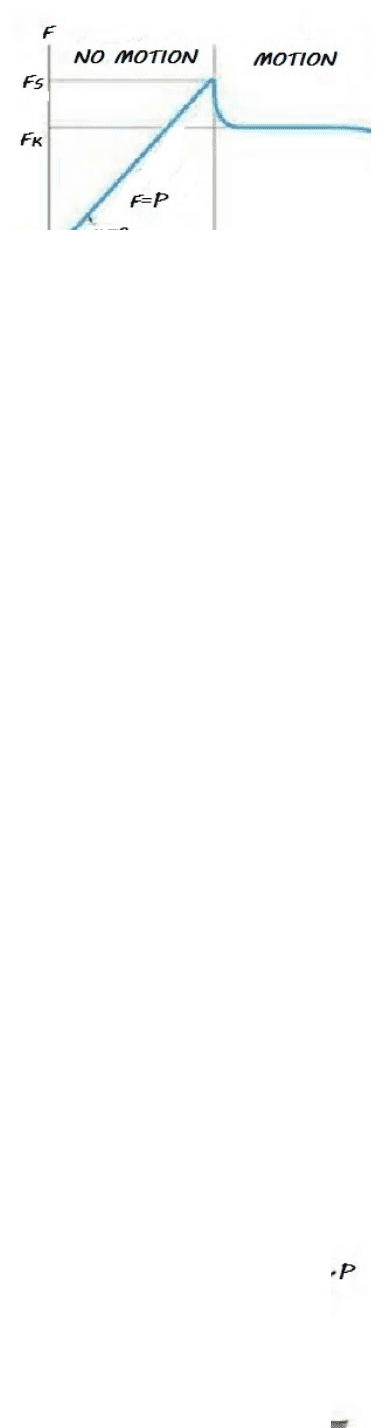# BUSTEC 101 Lecture 10: sheet 10 FRICTION

53 views4 pagesFriction
according to the figure:
1 .If th e force p is s m all, th e body will b e
still at rest(in eq uilibrium.(
So, f<µN, N is th e nor m al reaction.
2 .After p increa s ed to reach the value of f
s=µs N, the body is on
verge of slipping or about to slide, where fs is the static frictional
force,
µs is the static frictional coefficient.
3 .When p exc e e ds f
s , the frictional force reduced to the kinetic
frictional force fk=µkN where µk is the kinetic frictional coefficient.
As a consequence the body will move.
That m e a ns:
If f < µN th e body still in e quilibrium,
If fs=µs N the body in impending motion,
If fs>µs N the body in motion.
f.8.1. If th e p = 2 0 0 n, d et er mine th e frictional force d eveloped
b etwe en th e 50-kg crate a n d the ground. The coefficient of friction
µs is 0.3.
Ans, f= 160 n
F.8.2. Det er mine the minimu m force p to prevent th e 30-kg
rod AB from sliding, the contact surface at b is s mooth, whereas
Unlock document

This preview shows page 1 of the document.
Unlock all 4 pages and 3 million more documents.